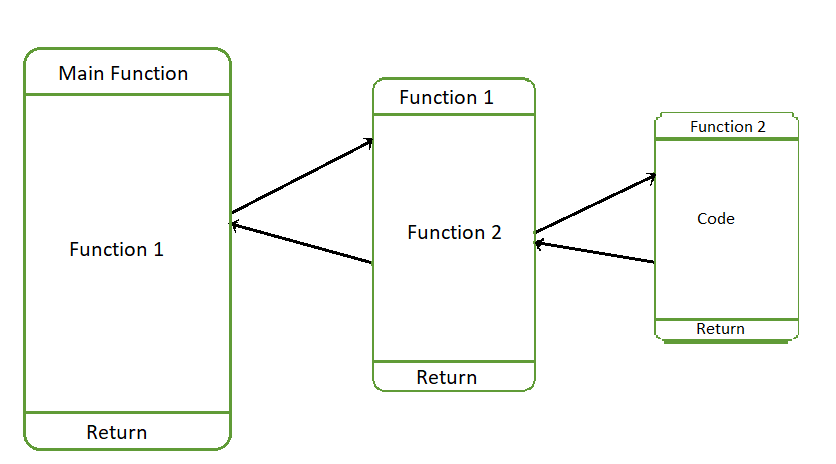# Python – Call function from another function

Prerequisite: Functions in Python

In Python, any written function can be called by another function. Note that this could be the most elegant way of breaking a problem into chunks of small problems. In this article, we will learn how can we call a defined function from another function with help of multiple examples.

Calling and Called Function ?
The Function which calls another Function is called Calling Function and function which is called by another Function is call Called Function.

How Function execution works ?
A stack data structure is used during the execution of the function calls. Whenever a function is invoked then the calling function is pushed into the stack and called function is executed. When the called function completes its execution and returns then the calling function is popped from the stack and executed. Calling Function execution will be completed only when called Function is execution completes.

In the below figure. The function call is made from the Main function to Function1, Now the state of the Main function is stored in Stack and execution of the Main function is continued when the Function 1 returns. The Fucntion1 Calls Function2 now the State of the Function1 is stored stack and execution of Function 1 will be continued when Function 2 returns.Consider the below Example of the function call. The Function `SumOfSquares `function calls the Function `Square `which returns the square of the number.

 `# Python code to demonstrate calling the  ` `# function from another function ` ` `  `def` `Square(X): ` `    ``# computes the Square of the given number  ` `    ``# and return to the caller function ` `    ``return` `(X ``*` `X) ` ` `  `def` `SumofSquares(Array, n): ` ` `  `    ``# Initialize variable Sum to 0. It stores the  ` `    ``# Total sum of squares of the array of elements ` `    ``Sum` `=` `0` `    ``for` `i ``in` `range``(n): ` ` `  `        ``# Square of Array[i] element is stored in SquaredValue ` `        ``SquaredValue ``=` `Square(Array[i]) ` ` `  `        ``# Cummulative sum is stored in Sum variable ` `        ``Sum` `+``=` `SquaredValue ` `    ``return` `Sum` ` `  `# Driver Function ` `Array ``=` `[``1``, ``2``, ``3``, ``4``, ``5``, ``6``, ``7``, ``8``, ``9``, ``10``] ` `n ``=` `len``(Array) ` ` `  `# Return value from the function  ` `# Sum of Squares is stored in Total ` `Total ``=` `SumofSquares(Array, n) ` `print``(``"Sum of the Square of List of Numbers:"``, Total) `

Output :

```Sum of the Square of List of Numbers: 385
```

Calling Function From another Function within Same class –

In the below example, the class method `Function1 `calls method `Function2 `from the class.

 `''' ` `Call a function from within another function ` `in the same class in Python  ` `'''` ` `  `class` `Main: ` ` `  `    ``# constructor of Main class ` `    ``def` `__init__(``self``): ` `        ``# Initialization of the Strings ` `        ``self``.String1 ``=``"Hello"` `        ``self``.String2 ``=``"World"` ` `  `    ``def` `Function1(``self``): ` `        ``# calling Function2 Method ` `        ``self``.Function2() ` `        ``print``(``"Function1 : "``, ``self``.String2) ` `        ``return`  ` `  `    ``def` `Function2(``self``): ` `        ``print``(``"Function2 : "``, ``self``.String1) ` `        ``return` ` `  `# Instance of Class Main ` `Object` `=` `Main() ` ` `  `# Calling Function1 ` `Object``.Function1() `

Output :

```Function2 :  Hello
Function1 :  World
```

Calling parent class Function from Child class Function –

Consider the below example the child class method invokes the parent class method. The child class inherits the attributes from the parent class.

 `# Python code to demonstrate calling parent class  ` `# method from the child class method ` ` `  `class` `Parent: ` ` `  `    ``# constructor of Parent class ` `    ``def` `__init__(``self``): ` `        ``# Initialization of the Strings ` `        ``self``.String1 ``=``"Hello"` `        ``self``.String2 ``=``"World"` ` `  `    ``def` `Function2(``self``): ` `        ``print``(``"Function2 : "``, ``self``.String1) ` `        ``return` ` `  `# Child class is inheriting from Parent class ` `class` `Child(Parent): ` ` `  `    ``def` `Function1(``self``): ` `        ``# calling Function2 Method in parent class  ` `        ``self``.Function2() ` `        ``print``(``"Function1 : "``, ``self``.String2) ` `        ``return`    ` `  `### Instance of Parent class ` `Object1 ``=` `Parent() ` ` `  `### Instance of Child class ` `Object2 ``=` `Child() ` ` `  `# Calling Function1 using Child class instance ` `Object2.Function1() `

Output :

```Function2 :  Hello
Function1 :  World
```

My Personal Notes arrow_drop_upCheck out this Author's contributed articles.

If you like GeeksforGeeks and would like to contribute, you can also write an article using contribute.geeksforgeeks.org or mail your article to contribute@geeksforgeeks.org. See your article appearing on the GeeksforGeeks main page and help other Geeks.

Please Improve this article if you find anything incorrect by clicking on the "Improve Article" button below.

Article Tags :

1

Please write to us at contribute@geeksforgeeks.org to report any issue with the above content.